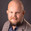# EQ1120 Discrete Time Signals and Systems 6.0 creditsLinear difference equations. characteristic equation, discrete-time Fourier transform, single and double sided Z-transform, discrete-time systems and system properties, impulse  response, convolution, transfer function, frequency function, sinus in sinus out, poles and zeroes, stability criteria for discrete time systems, sampling and reconstruction by pules amplitude modulation, sampling theorem, system realizations in Matlab.

The aim is to provide basic knowledge about discrete-time signals and systems, how they can be described and analyzed by using difference equations and transform methods, and be implemented in software like Matlab.Linear difference equations. characteristic equation, discrete-time Fourier transform, single and double sided Z-transform, discrete-time systems and system properties, impulse  response, convolution, transfer function, frequency function, sinus in sinus out, poles and zeroes, stability criteria for discrete time systems, sampling and reconstruction by pules amplitude modulation, sampling theorem, system realizations in Matlab.

The aim is to provide basic knowledge about discrete-time signals and systems, how they can be described and analyzed by using difference equations and transform methods, and be implemented in software like Matlab.

### Choose semester and course offering

Choose semester and course offering to see information from the correct course syllabus and course offering.

Headings with content from the Course syllabus EQ1120 (Autumn 2021–) are denoted with an asterisk ( )

## Content and learning outcomes

### Course contents

Linear difference equations, characteristic equation, Discrete-time Fourier transfom, one- and double-sided z-transform, discrete-time systems and system properties, impulse response and convolution, transfer and frequency function, sine-in-sine-out, poles and zeros, stability criteria for discrete-time systems, sampling and reconstruction by pulse amplitude modulation, the sampling theorem, system realizations in Matlab.

### Intended learning outcomes

The course gives basic knowledge of discrete-time signals and systems and how these can be described and analysed by using difference equations and transform methods and be implemented in software such as Matlab.

After approval in the course the student should be able to

• solve simple linear difference equations with and without initial conditions
• be able to implement and simulate discrete-time systems in Matlab numerically
• give an account of the definition and the meaning of system properties such as linearity, time invariance, causality and stability
• describe linear and time invariant systems by means of their impulse response, and through convolution be able to calculate the output signal for a system given an input signal
• calculate the discrete-time Fourier transform and its inverse for given signals based on definition and general properties for transforms
• calculate the double- and the one-sided z-transform for a given signal, use these transforms to solve difference equations and output signals of systems and through partial fraction decomposition and tables be able to recover the time domain description of solutions and output signals
• interpret the placements of poles and zeros in terms of the frequency domain properties of the discrete-time system
• decide the stability of causal LTI systems through the placement of the poles
• give an account of the concepts sampling and reconstruction through pulse amplitude modulation
• describe folding in the time and frequency domain
• describe the spectrum of a sampled signal in terms of the spectrum of the original signal and give an account of the importance of the Nyquist criterion (the sampling theorem)
• calculate the total transfer function for a sampled system
• describe in speech and writing how a system solves a given signal processing task

For higher grades, the student should also be able to

• demonstrate an understanding of the relationships between the different descriptions of discrete-time systems and their properties in the time and  transform domain
• be able to choose appropriate solution methods for a given problem
• combine different concepts and methods from the course and apply them on more complex mathematical and technical problem formulations

### Course disposition

No information inserted

## Literature and preparations

### Specific prerequisites

Knowledge in one variable calculus, 7.5 higher education credits, equivalent to completed course SF1625.

Active participation in a course offering where the final examination is not yet reported in LADOK is considered equivalent to completion of the course. Registering for a course is counted as active participation. The term 'final examination' encompasses both the regular examination and the first re-examination.

### Recommended prerequisites

SF1624 Algebra and geometry

EQ1110 Continuous time signals and systems

### Equipment

No information inserted

### Literature

No information inserted

## Examination and completion

If the course is discontinued, students may request to be examined during the following two academic years.

A, B, C, D, E, FX, F

### Examination

• PRO1 - Project Work, 1.0 credits, grading scale: P, F
• PRO2 - Project Work, 1.0 credits, grading scale: A, B, C, D, E, FX, F
• TEN1 - Exam, 4.0 credits, grading scale: A, B, C, D, E, FX, F

Based on recommendation from KTH’s coordinator for disabilities, the examiner will decide how to adapt an examination for students with documented disability.

The examiner may apply another examination format when re-examining individual students.

### Other requirements for final grade

Approved examination in all course components.

Final mark is combined 80% based on TEN1 and 20% based on PRO2

### Opportunity to complete the requirements via supplementary examination

No information inserted

### Opportunity to raise an approved grade via renewed examination

No information inserted

### ExaminerJoakim Jaldén

### Ethical approach

• All members of a group are responsible for the group's work.
• In any assessment, every student shall honestly disclose any help received and sources used.
• In an oral assessment, every student shall be able to present and answer questions about the entire assignment and solution.

## Further information

### Course web

Further information about the course can be found on the Course web at the link below. Information on the Course web will later be moved to this site.

Course web EQ1120

### Offered by

EECS/Intelligent Systems

Technology

First cycle